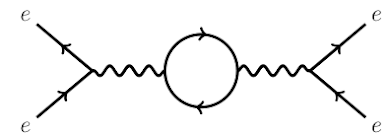### JMP #58, 4: path integrals and friendsThe path integral formulation of quantum mechanics replaces the single, classical trajectory of a system with the sum over an infinity of quantum possible trajectories. To compute this sum is used a functional integral. The most famous interpretation is dued by Richard Feynman. In an Euclidean spacetime we speak about Euclidean path integral:
Bernardo, R. C. S., & Esguerra, J. P. H. (2017). Euclidean path integral formalism in deformed space with minimum measurable length. Journal of Mathematical Physics, 58(4), 042103. doi:10.1063/1.4979797
We study time-evolution at the quantum level by developing the Euclidean path-integral approach for the general case where there exists a minimum measurable length. We derive an expression for the momentum-space propagator which turns out to be consistent with recently developed $\beta$-canonical transformation. We also construct the propagator for maximal localization which corresponds to the amplitude that a state which is maximally localized at location $\xi'$ propagates to a state which is maximally localized at location $\xi"$ in a given time. Our expression for the momentum-space propagator and the propagator for maximal localization is valid for any form of time-independent Hamiltonian. The nonrelativistic free particle, particle in a linear potential, and the harmonic oscillator are discussed as examples.
Other papers from JMP #58, 4, follows:
Okołów, A. (2017). Kinematic projective quantum states for loop quantum gravity coupled to tensor fields. Journal of Mathematical Physics, 58(4), 042302. doi:10.1063/1.4980014 (sci-hub)
We present a construction of kinematic quantum states for theories of tensor fields of an arbitrary sort. The construction is based on projective techniques by Kijowski. Applying projective quantum states for Loop Quantum Gravity (LQG) obtained by Lanéry and Thiemann we construct quantum states for LQG coupled to tensor fields.
Almeida, C. R., Batista, A. B., Fabris, J. C., & Moniz, P. V. (2017). Quantum cosmology of scalar-tensor theories and self-adjointness. Journal of Mathematical Physics, 58(4), 042301. doi:10.1063/1.4979537 (arXiv)
In this paper, the problem of the self-adjointness for the case of a quantum mini-superspace Hamiltonian retrieved from a Brans-Dicke action is investigated. Our matter content is presented in terms of a perfect fluid, onto which Schutz’s formalism will be applied. We use the von Neumann theorem and the similarity with the Laplacian operator in one of the variables to determine the cases where the Hamiltonian is self-adjoint and if it admits self-adjoint extensions. For the latter, we study which extension is physically more suitable.
Dudnikova, T. V. (2017). On convergence to equilibrium for one-dimensional chain of harmonic oscillators on the half-line. Journal of Mathematical Physics, 58(4), 043301. doi:10.1063/1.4979629 (arXiv)
The mixing boundary-value problem for infinite one-dimensional chain of harmonic oscillators on the half-line is considered. The large time asymptotic behavior of solutions is obtained. The initial data of the system are supposed to be a random function which has some mixing properties.
Li, Q., & Wu, X. (2017). Existence, multiplicity, and concentration of solutions for generalized quasilinear Schrödinger equations with critical growth. Journal of Mathematical Physics, 58(4), 041501. doi:10.1063/1.4982035 (sci-hub)
In this paper, we study the following generalized quasilinear Schrödinger equations (...). Under some suitable conditions, we study the existence, multiplicity, and concentration of solutions by the variational methods.
Exner, P., & Lipovský, J. (2017). Pseudo-orbit approach to trajectories of resonances in quantum graphs with general vertex coupling: Fermi rule and high-energy asymptotics. Journal of Mathematical Physics, 58(4), 042101. doi:10.1063/1.4979048 (sci-hub)
The aim of the paper is to investigate resonances in quantum graphs with a general self-adjoint coupling in the vertices and their trajectories with respect to varying edge lengths. We derive formulae determining the Taylor expansion of the resonance pole position up to the second order, which represent, in particular, a counterpart to the Fermi rule derived recently by Lee and Zworski for graphs with the standard coupling. Furthermore, we discuss the asymptotic behavior of the resonances in the high-energy regime in the situation where the leads are attached through $\delta$ or $\delta'_s$ conditions, and we prove that in the case of $\delta'_s$ coupling the resonances approach to the real axis with the increasing real parts as $\mathscr{O} \left ( (\text{Re} \, k)^{−2} \right )$.